×Professor Switkes

Review of Functions

Slide Duration:

Section 1: Overview of Functions
Review of Functions

26m 29s

Intro
0:00
What is a Function
0:10
Domain and Range
0:21
Vertical Line Test
0:31
Example: Vertical Line Test
0:47
Function Examples
1:57
Example: Squared
2:10
Example: Natural Log
2:41
Example: Exponential
3:21
Example: Not Function
3:54
Odd and Even Functions
4:39
Example: Even Function
5:10
Example: Odd Function
5:53
Odd and Even Examples
6:48
Odd Function
6:55
Even Function
8:43
Increasing and Decreasing Functions
10:15
Example: Increasing
10:42
Example: Decreasing
10:55
Increasing and Decreasing Examples
11:41
Example: Increasing
11:48
Example: Decreasing
12:33
Types of Functions
13:32
Polynomials
13:45
Powers
14:06
Trigonometric
14:34
Rational
14:50
Exponential
15:13
Logarithmic
15:29
Lecture Example 1
15:55
Lecture Example 2
17:51
-1
-2
Compositions of Functions

12m 29s

Intro
0:00
Compositions
0:09
Alternative Notation
0:32
Three Functions
0:47
Lecture Example 1
1:19
Lecture Example 2
3:25
Lecture Example 3
6:45
-1
-2
Section 2: Limits
Average and Instantaneous Rates of Change

20m 59s

Intro
0:00
Rates of Change
0:11
Average Rate of Change
0:21
Instantaneous Rate of Change
0:33
Slope of the Secant Line
0:46
Slope of the Tangent Line
1:00
Lecture Example 1
1:14
Lecture Example 2
6:36
Lecture Example 3
11:30
-1
-2
Limit Investigations

22m 37s

Intro
0:00
What is a Limit?
0:10
Lecture Example 1
0:56
Lecture Example 2
5:28
Lecture Example 3
9:27
-1
-2
Algebraic Evaluation of Limits

28m 19s

Intro
0:00
Evaluating Limits
0:09
Lecture Example 1
1:06
Lecture Example 2
5:16
Lecture Example 3
8:15
Lecture Example 4
12:58
-1
-2
Formal Definition of a Limit

23m 39s

Intro
0:00
Formal Definition
0:13
Template
0:55
Epsilon and Delta
1:24
Lecture Example 1
1:40
Lecture Example 2
9:20
-1
-2
Continuity and the Intermediate Value Theorem

19m 9s

Intro
0:00
Continuity
0:13
Continuous
0:16
Discontinuous
0:37
Intermediate Value Theorem
0:52
Example
1:22
Lecture Example 1
2:58
Lecture Example 2
9:02
-1
-2
Section 3: Derivatives, part 1
Limit Definition of the Derivative

22m 52s

Intro
0:00
Limit Definition of the Derivative
0:11
Three Versions
0:13
Lecture Example 1
1:02
Lecture Example 2
4:33
Lecture Example 3
6:49
Lecture Example 4
10:11
-1
-2
The Power Rule

26m 1s

Intro
0:00
Power Rule of Differentiation
0:14
Power Rule with Constant
0:41
Sum/Difference
1:15
Lecture Example 1
1:59
Lecture Example 2
6:48
Lecture Example 3
11:22
-1
-2
The Product Rule

14m 54s

Statement of the Product Rule
0:08
Lecture Example 1
0:41
Lecture Example 2
2:27
Lecture Example 3
5:03
-1
-2
The Quotient Rule

19m 17s

Intro
0:00
Statement of the Quotient Rule
0:07
Carrying out the Differentiation
0:23
Quotient Rule in Words
1:00
Lecture Example 1
1:19
Lecture Example 2
4:23
Lecture Example 3
8:00
-1
-2
Applications of Rates of Change

17m 43s

Intro
0:00
Rates of Change
0:11
Lecture Example 1
0:44
Lecture Example 2
5:16
Lecture Example 3
7:38
-1
-2
Trigonometric Derivatives

26m 58s

Intro
0:00
Six Basic Trigonometric Functions
0:11
Patterns
0:47
Lecture Example 1
1:18
Lecture Example 2
7:38
Lecture Example 3
12:15
Lecture Example 4
14:25
-1
-2
The Chain Rule

23m 47s

Intro
0:00
Statement of the Chain Rule
0:09
Chain Rule for Three Functions
0:27
Lecture Example 1
1:00
Lecture Example 2
4:34
Lecture Example 3
7:23
-1
-2
Inverse Trigonometric Functions

27m 5s

Intro
0:00
Six Basic Inverse Trigonometric Functions
0:10
Lecture Example 1
1:11
Lecture Example 2
8:53
Lecture Example 3
12:37
-1
-2
Equation of a Tangent Line

15m 52s

Intro
0:00
Point Slope Form
0:10
Lecture Example 1
0:47
Lecture Example 2
3:15
Lecture Example 3
6:10
-1
-2
Section 4: Derivatives, part 2
Implicit Differentiation

30m 5s

Intro
0:00
Purpose
0:09
Implicit Function
0:20
Lecture Example 1
0:32
Lecture Example 2
7:14
Lecture Example 3
11:22
Lecture Example 4
16:43
-1
-2
Higher Derivatives

13m 16s

Intro
0:00
Notation
0:08
First Type
0:19
Second Type
0:54
Lecture Example 1
1:41
Lecture Example 2
3:15
Lecture Example 3
4:57
-1
-2
Logarithmic and Exponential Function Derivatives

17m 42s

Intro
0:00
Essential Equations
0:12
Lecture Example 1
1:34
Lecture Example 2
2:48
Lecture Example 3
5:54
-1
-2
Hyperbolic Trigonometric Function Derivatives

14m 30s

Intro
0:00
Essential Equations
0:15
Six Basic Hyperbolic Trigc Functions
0:32
Six Basic Inverse Hyperbolic Trig Functions
1:21
Lecture Example 1
1:48
Lecture Example 2
3:45
Lecture Example 3
7:09
-1
-2
Related Rates

29m 5s

Intro
0:00
What Are Related Rates?
0:08
Lecture Example 1
0:35
Lecture Example 2
5:25
Lecture Example 3
11:54
-1
-2
Linear Approximation

23m 52s

Intro
0:00
Essential Equations
0:09
Linear Approximation (Tangent Line)
0:18
Example: Graph
1:18
Differential (df)
2:06
Delta F
5:10
Lecture Example 1
6:38
Lecture Example 2
11:53
Lecture Example 3
15:54
-1
-2
Section 5: Application of Derivatives
Absolute Minima and Maxima

18m 57s

Intro
0:00
Minimums and Maximums
0:09
Absolute Minima and Maxima (Extrema)
0:53
Critical Points
1:25
Lecture Example 1
2:58
Lecture Example 2
6:57
Lecture Example 3
10:02
-1
-2
Mean Value Theorem and Rolle's Theorem

20m

Intro
0:00
Theorems
0:09
Mean Value Theorem
0:13
Graphical Explanation
0:36
Rolle's Theorem
2:06
Graphical Explanation
2:28
Lecture Example 1
3:36
Lecture Example 2
6:33
Lecture Example 3
9:32
-1
-2
First Derivative Test, Second Derivative Test

27m 11s

Intro
0:00
Local Minimum and Local Maximum
0:14
Example
1:01
First and Second Derivative Test
1:26
First Derivative Test
1:36
Example
2:00
Second Derivative Test (Concavity)
2:58
Example: Concave Down
3:15
Example: Concave Up
3:54
Inconclusive
4:19
Lecture Example 1
5:23
Lecture Example 2
12:03
Lecture Example 3
15:54
-1
-2
L'Hopital's Rule

23m 9s

Intro
0:00
Using L'Hopital's Rule
0:09
Informal Definition
0:34
Lecture Example 1
1:27
Lecture Example 2
4:00
Lecture Example 3
5:40
Lecture Example 4
9:38
-1
-2
Curve Sketching

40m 16s

Intro
0:00
Collecting Information
0:15
Domain and Range
0:17
Intercepts
0:21
Symmetry Properties (Even/Odd/Periodic)
0:33
Asymptotes (Vertical/Horizontal/Slant)
0:45
Critical Points
1:15
Increasing/Decreasing Intervals
1:24
Inflection Points
1:38
Concave Up/Down
1:52
Maxima/Minima
2:03
Lecture Example 1
2:58
Lecture Example 2
10:52
Lecture Example 3
17:55
-1
-2
Applied Optimization

25m 37s

Intro
0:00
Real World Problems
0:08
Sketch
0:11
Interval
0:20
Rewrite in One Variable
0:26
Maximum or Minimum
0:34
Critical Points
0:42
Optimal Result
0:52
Lecture Example 1
1:05
Lecture Example 2
6:12
Lecture Example 3
13:31
-1
-2
Newton's Method

25m 13s

Intro
0:00
Approximating Using Newton's Method
0:10
Good Guesses for Convergence
0:32
Lecture Example 1
0:49
Lecture Example 2
4:21
Lecture Example 3
7:59
-1
-2
Section 6: Integrals
Approximating Areas and Distances

36m 50s

Intro
0:00
Three Approximations
0:12
Right Endpoint, Left Endpoint, Midpoint
0:22
Formulas
1:05
Velocity and Distance
1:35
Lecture Example 1
2:28
Lecture Example 2
12:10
Lecture Example 3
19:43
-1
-2
Riemann Sums, Definite Integrals, Fundamental Theorem of Calculus

22m 2s

Intro
0:00
Important Equations
0:22
Riemann Sum
0:28
Integral
1:58
Integrand
2:35
Limits of Integration (Upper Limit, Lower Limit)
2:43
Other Equations
3:05
Fundamental Theorem of Calculus
4:00
Lecture Example 1
5:04
Lecture Example 2
10:43
Lecture Example 3
13:52
-1
-2
Substitution Method for Integration

23m 19s

Intro
0:00
U-Substitution
0:13
Important Equations
0:30
Purpose
0:36
Lecture Example 1
1:30
Lecture Example 2
6:17
Lecture Example 3
9:00
Lecture Example 4
11:24
-1
-2
Section 7: Application of Integrals, part 1
Area Between Curves

19m 59s

Intro
0:00
Area Between Two Curves
0:12
Graphic Description
0:34
Lecture Example 1
1:44
Lecture Example 2
5:39
Lecture Example 3
8:45
-1
-2
Volume by Method of Disks and Washers

24m 22s

Intro
0:00
Important Equations
0:16
Equation 1: Rotation about x-axis (disks)
0:27
Equation 2: Two curves about x-axis (washers)
3:38
5:31
Lecture Example 1
6:05
Lecture Example 2
8:28
Lecture Example 3
11:55
-1
-2
Volume by Method of Cylindrical Shells

30m 29s

Intro
0:00
Important Equations
0:50
1:04
Equation 2: Rotation about y-axis (2 curves)
7:34
8:15
Lecture Example 1
8:57
Lecture Example 2
14:26
Lecture Example 3
18:15
-1
-2
Average Value of a Function

16m 31s

Intro
0:00
Important Equations
0:11
Origin of Formula
0:34
Lecture Example 1
2:51
Lecture Example 2
5:30
Lecture Example 3
8:13
-1
-2
Section 8: Extra
Graphs of f, f', f''

23m 58s

Intro
0:00
Slope Function of f(x)
0:41
Slope is Zero
0:53
Slope is Positive
1:03
Slope is Negative
1:13
Slope Function of f'(x)
1:31
Slope is Zero
1:42
Slope is Positive
1:48
Slope is Negative
1:54
Lecture Example 1
2:23
Lecture Example 2
8:06
Lecture Example 3
12:36
-1
-2
Slope Fields for Differential Equations

18m 32s

Intro
0:00
Things to Remember
0:13
Graphic Description
0:42
Lecture Example 1
1:44
Lecture Example 2
6:59
Lecture Example 3
9:46
-1
-2
Separable Differential Equations

17m 4s

Intro
0:00
Differential Equations
0:10
Focus on Exponential Growth/Decay
0:27
Separating Variables
0:47
Lecture Example 1
1:35
Lecture Example 2
6:41
Lecture Example 3
9:36
-1
-2

• ## Related Books

### Review of Functions

• These are review topics from algebra and pre-calculus – it would be good to just briefly “brush up” on these things!
• As you go through calculus, it will be important to use the correct terminology for the various terms associated with functions – clear mathematical communication is important!

### Review of Functions

Simplify tanx cscx
• y = tanx cscx
• = [sinx/cosx] [1/sinx]
= [1/cosx] = secx
Simplify cscx cosx
• y = cscx cosx
• = [1/sinx] cosx
• = [cosx/sinx]
=cotx
Simplify [4x sinx/(x2 cos3x)]
• y = [4x sinx/(x2 cos3x)]
• = [4x/(x2)] [sinx/cos3x]
and cannot be combined because their arguments differ. The arguments must be the same to rewrite trig functions. 0Find the domain and range of
• sin and cos are periodic functions, meaning the outputs will repeat once the input is shifted over a full period. The unit circle is a good way to see this. No matter what real numbers we put into the function, the same pattern repeats. The output never exceeds 1 or goes below -1
•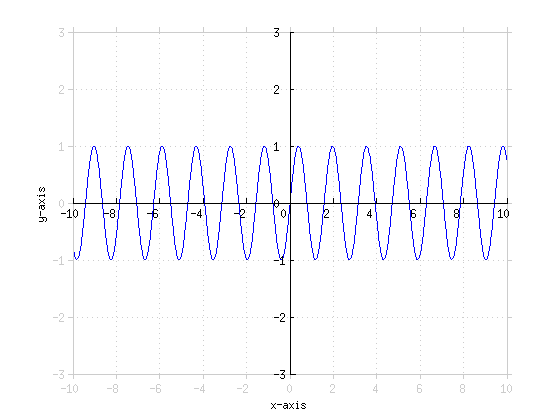Domain (), Range [-1, 1] 0Find the domain and range of
This is similar to the previous problem, but it's a bit trickier because it introduces zero into the denominator. The function is said to be undefined where the input results in a zero in the denominator. For this function, we won't have any problems when the denominator is not zero. So the domain involves any x when is not zero. is zero at any multiple of , including zero. Now, we're dividing by really tiny numbers (approaching zero). An integer divided by a very small number, is a very large number. Domain is all real numbers, but cannot equal any multiple of . where n is any integer. Range 0Graph
• This is very similar to the regular cos graph, except that its period is changed, or quished." Its usual period of is now .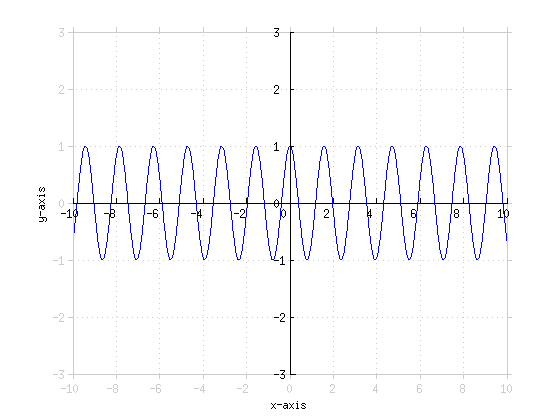0Graph
This is similar to the regular sin graph, but it is shifted UP by one unit.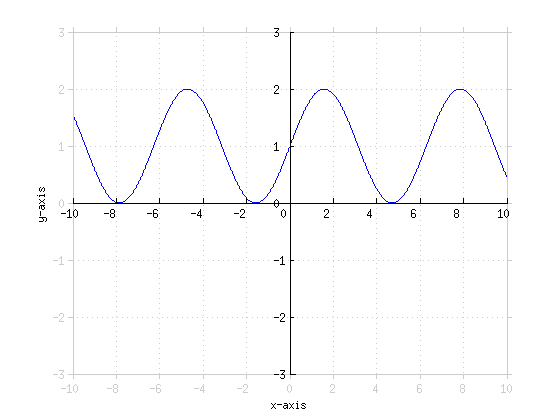0Graph
• is very different from . Radians are used unless otherwise specified. By convention, the variable typically implies use of degrees).
• has a period of , meaning that going units in x in either direction will return the same result
• etc...
• so this is the same graph as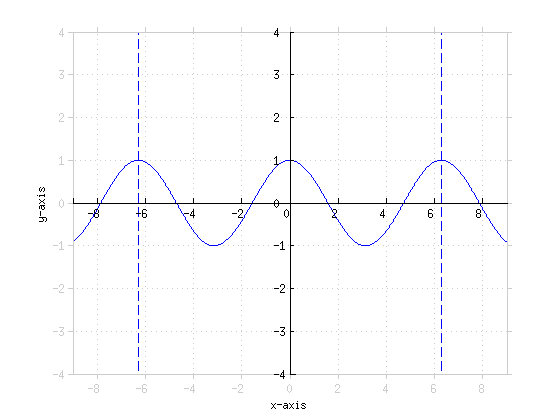0Graph
• The number out front here determines the amplitude. In this case, it's 3. So from minimum to maximum, this graph will cover 6 units total. Multiplying the x here by 2 changes the period from to .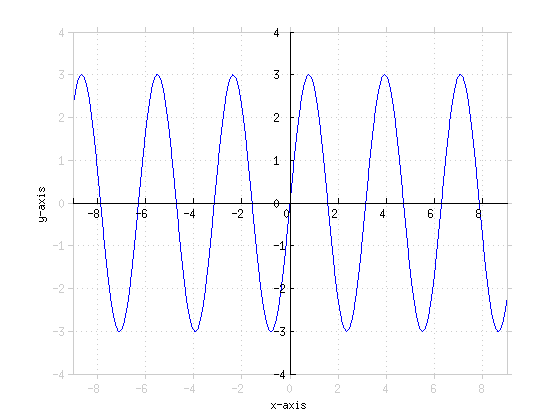0Graph
• The positive 4 there moves the graph over 4 units to the left. The -1 in that location means the graph is shifted down 1. This is the same graph as the previous problem, but shifted down 1 and 4 to the left.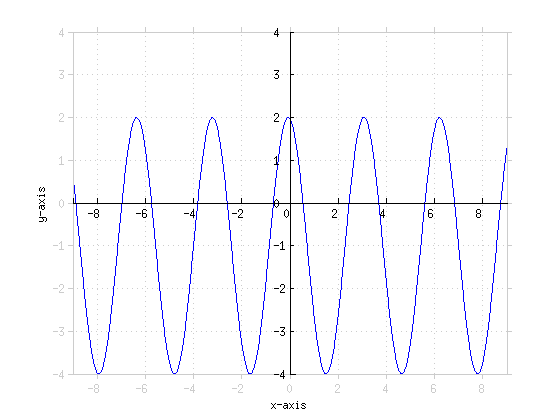0Simplify
• This is equivalent to , which would give an exponent of , or
0Rewrite
0Rewrite
0Combine the exponents of
0Combine the exponents of
0Simplify
0Simplify
0Solve for x,
0Solve for x,
0Prove
• ADVANCED NOTE: This is the exponential expression of the trig identity
0Given , find
0Solve for x,
0Simplify
0Solve for x,
x = 1 0Solve for y,
0Solve for x,
0Solve for x,
0Simplify
Here, the base is irrelevant. 0Solve for ,
• The second solution, -9, would be outside of the domain of . For , x must be greater than 0. So
0Prove
• Let and
0Find the roots of
0Find the roots of
Two identical roots, 0Find the asymptote(s) of
We already found the roots on the previous problems. The asymptote is where the function is undefined. Here, it's when the denominator is equal to zero. We know that the denominator is equal to zero when . The function goes to positive infinity when approaching the root () from the positive direction. The function also goes to positive infinity when approaching the root from the negative direction (The degree of the polynomial in the denominator is even). 0Graph
• We already determined the asymptote behavior, what about the edge behavior? As x gets very large and positve, y approaches zero. As x gets very large and negative, y also approaches zero from the positive side.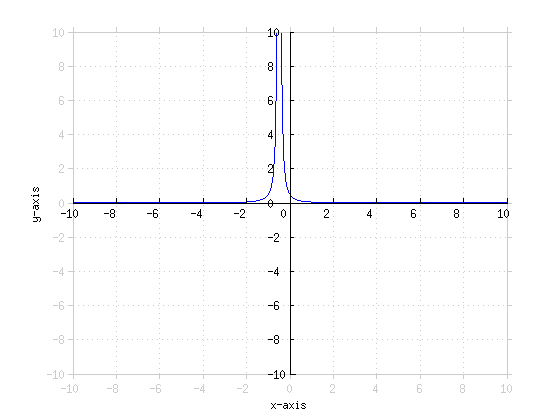0Find the asymptote(s) of
The function is undefined at x = 0. The function goes to positive infinity when approaching zero from the positive direction. The function goes to negative infinity when approaching zero from the negative direction (Remember, the polynomial in the denominator is of odd degree). 0Find the asymptote(s) of
• . The function is undefined when . when
There are an infinite number of asymptotes for and those asymptotes are at odd integer multiples of 0Graph
• This is similar to the graph of , but it is shifted 5 to the left.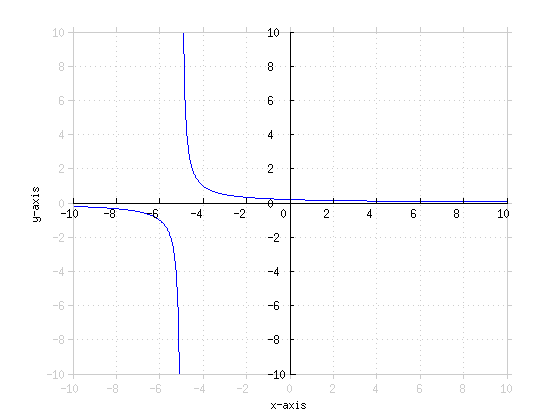0Graph
• Even degree polynomial in the denominator. As x gets very large in either direction, y approaches zero. There are two asymptotes here, -2 and 2. That gives us 4 different cases for asymptotic behavior. Approaching -2 from the left, Approaching -2 from the right, approaching 2 from the left, and approaching 2 from the right. Plugging in values to test for these cases.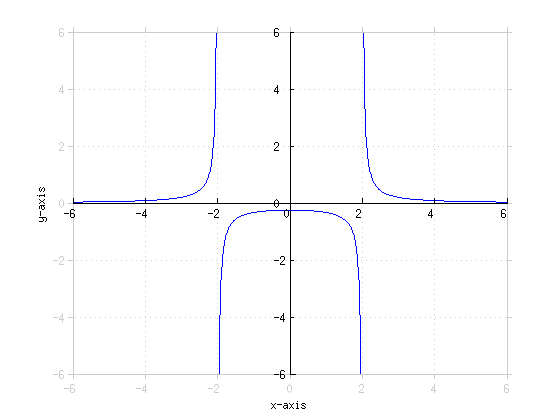0Graph
• Asymptotes are at same locations as previous problem, so we can find the asymptote behavior in a similar fashion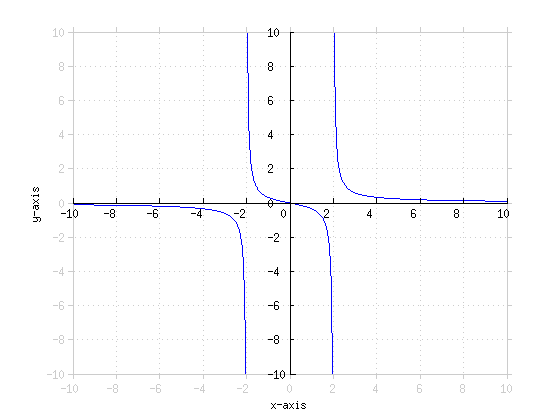0Graph
• Remember the properties of . Domain , , and is an increasing function. As x gets larger, f(x) approaches zero and f(x) has an asymptote at x = 1.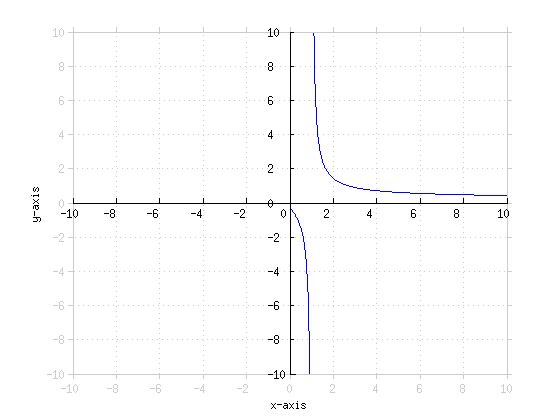*These practice questions are only helpful when you work on them offline on a piece of paper and then use the solution steps function to check your answer.

### Review of Functions

Lecture Slides are screen-captured images of important points in the lecture. Students can download and print out these lecture slide images to do practice problems as well as take notes while watching the lecture.

• Intro 0:00
• What is a Function 0:10
• Domain and Range
• Vertical Line Test
• Example: Vertical Line Test
• Function Examples 1:57
• Example: Squared
• Example: Natural Log
• Example: Exponential
• Example: Not Function
• Odd and Even Functions 4:39
• Example: Even Function
• Example: Odd Function
• Odd and Even Examples 6:48
• Odd Function
• Even Function
• Increasing and Decreasing Functions 10:15
• Example: Increasing
• Example: Decreasing
• Increasing and Decreasing Examples 11:41
• Example: Increasing
• Example: Decreasing
• Types of Functions 13:32
• Polynomials
• Powers
• Trigonometric
• Rational
• Exponential
• Logarithmic
• Lecture Example 1 15:55
• Lecture Example 2 17:51

OR

### Start Learning Now

Our free lessons will get you started (Adobe Flash® required).